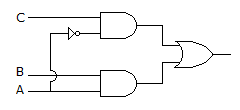# Electronics and Communication Engineering - Digital Electronics

21.

For the logic circuit of the given figure the simplified Boolean expression isA. Y = AB + A C B. Y = (AB) (A C) C. Y = ABC D. Y = A B + A C

Explanation:

No answer description available for this question. Let us discuss.

22.

In two level logic, logic race does not occur.

 A. True B. False